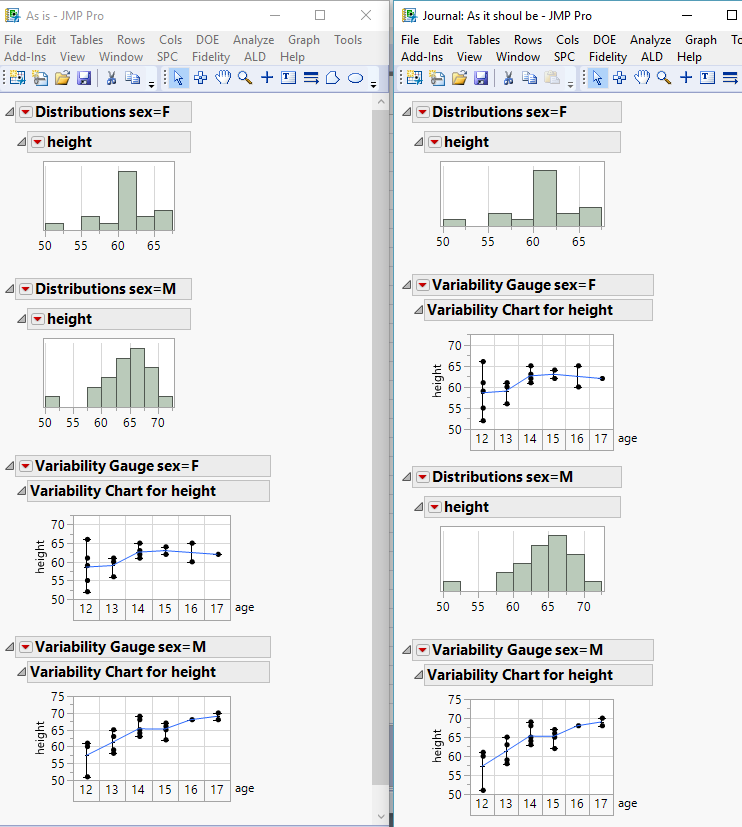Choose Language Hide Translation Bar
Highlighted

## Rearranging two platforms created with BY

Hello,

Left side is the regular sequence if I add Distribution with BY and then Variability with BY to a window.

Is there some way to get the sequence as is in the right side?

The solution have to be in JSL and without looping but with using BY option.

Thanks.1 ACCEPTED SOLUTION

Accepted Solutions
Highlighted

## Re: Rearranging two platforms created with BY

Here is the script, rewritten to make the Platforms Live

``````Names Default To Here( 1 );
dt = Open( "\$SAMPLE_DATA/big class.jmp" );

Summarize( dt, groupBy = by( :sex ) );

NW = New Window( "The Output", vlb = V List Box() );

For( i = 1, i <= N Items( groupBy ), i++,
vlb << append(
Eval(
Substitute(
Expr(
dis =
Distribution(
Continuous Distribution(
Column( :height ),
Quantiles( 0 ),
Summary Statistics(
0
),
Horizontal Layout( 1 ),
Vertical( 0 )
),
where( :sex == __where__ )
);

var =
Variability Chart(
Y( :height ),
X( :age ),
Std Dev Chart( 0 ),
where( :sex == __where__ )
);
),
Expr( __where__ ), groupBy[i]
)
)
)
);``````
Jim
3 REPLIES 3
Highlighted

## Re: Rearranging two platforms created with BY

Here is a very simple script, showing one way to do this

``````names default to here(1);
dt = open("\$SAMPLE_DATA/big class.jmp");
dis = Distribution(
Continuous Distribution(invisible,
Column( :height ),
Quantiles( 0 ),
Summary Statistics( 0 ),
Horizontal Layout( 1 ),
Vertical( 0 )
),
by( :sex )
);

var = Variability Chart(invisible,
Y( :height ),
X( :age ),
Std Dev Chart( 0 ),
by(:sex)
);

new window("together",
vlb=vlistbox()
);

vlb << append(report(dis)[OutlineBox(1)]);

vlb << append(report(var)[OutlineBox(1)]);

vlb << append(report(dis)[OutlineBox(1)]);

vlb << append(report(var)[OutlineBox(1)]);

var << close window;
dis << close window;``````
Jim
Highlighted

## Re: Rearranging two platforms created with BY

Thank you Jim.

1. I need the graphs "alive", not reports only.

2. If I have many groups, I need loop. I am looking for solution without looping.

Something during creation of the platforms may be...

Highlighted

## Re: Rearranging two platforms created with BY

Here is the script, rewritten to make the Platforms Live

``````Names Default To Here( 1 );
dt = Open( "\$SAMPLE_DATA/big class.jmp" );

Summarize( dt, groupBy = by( :sex ) );

NW = New Window( "The Output", vlb = V List Box() );

For( i = 1, i <= N Items( groupBy ), i++,
vlb << append(
Eval(
Substitute(
Expr(
dis =
Distribution(
Continuous Distribution(
Column( :height ),
Quantiles( 0 ),
Summary Statistics(
0
),
Horizontal Layout( 1 ),
Vertical( 0 )
),
where( :sex == __where__ )
);

var =
Variability Chart(
Y( :height ),
X( :age ),
Std Dev Chart( 0 ),
where( :sex == __where__ )
);
),
Expr( __where__ ), groupBy[i]
)
)
)
);``````
Jim
Article Labels